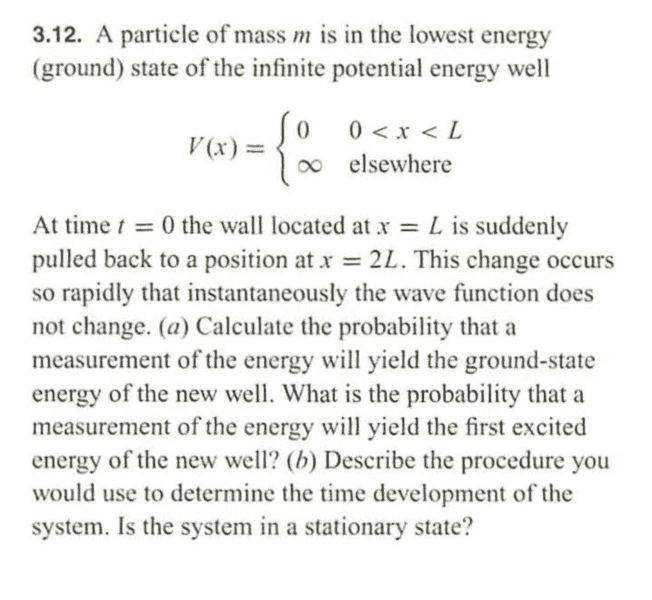# Evolution of a particle in a well

• MikeyArey
In summary, the new well does not have an energy eigenstate of the old well, so there is no answer to the question about whether the wave function changes.f

## Homework Statement

I posted a picture of the question https://imgur.com/a/8byywYL

## Homework Equations

P = $$(<\psi_{n}|\psi_{o}>)^2$$

## The Attempt at a Solution

I am guessing that I compute(denote by ##\psi_{o}## the ground state of the old well and by ##\psi_{n}## the ground state of the new well) $$(<\psi_{n}|\psi_{o}>)^2$$ I think this answer is meaningless because the system itself changed. The well is no longer the same. How can we take a state of one system and take its inner product with a state from a completely different system? I have a feeling that the answer to the first part of the question is zero, because in the question it is said "the wave function does not change". I do not understand how the artificial nature of this question can give any meaningful answer.

Maybe since the question does not specify a certain point in time, the answer is the same for all times, and is thus thatfor initial time.

Also, the state is stationary if it is an energy eigenvector, but how can we know if it is one?#### Attachments

Let's say we have a particle in an infinite well, and let's also say it is in the ground state. What happens when we make the well bigger(increase its length)? Does the particle stay in the same state or does it become the ground state of the new well?

Does the new state of the particle depend on whether the ground state of the initial well is an energy eigenstate of the new well(so the answer would depend on the length)?

What if the old ground state is no longer an energy eigenstate of the new well?

Assume for concreteness that the inital (1D)well is from x=0 to x=L and the new well is from x=0 to x=2L. We simply pull the right wall of the well to the right very quickly.

The particle is initially in the same state, which is no longer an eigenstate of the new well. You can represent the original eigenstate as a linear combination of eigenstates of the new well. The state then evolves over time according to the Schrodinger equation.

The particle is initially in the same state, which is no longer an eigenstate of the new well. You can represent the original eigenstate as a linear combination of eigenstates of the new well. The state then evolves over time according to the Schrodinger equation.
So it is impossible for the particle to stay in the ground state of the old potential?

You could think of the classical analogy. A particle is bouncing between two walls, when one wall is moved. The particle doesn't change its behaviour until it reaches where the wall had been. And then it settles into a different oscillation appropriate to the new conditions.

So it is impossible for the particle to stay in the ground state of the old potential?
Wells of different widths have no eigenstates in common. For the reason that the width of the well is a parameter in the sine/cosine functions.

•MikeyArey
You could think of the classical analogy. A particle is bouncing between two walls, when one wall is moved. The particle doesn't change its behaviour until it reaches where the wall had been. And then it settles into a different oscillation appropriate to the new conditions.
So how do I compute the probability that the particle will have ground state energy? Or first excited state?

Wells of different widths have no eigenstates in common. For the reason that the width of the well is a parameter in the sine/cosine functions.
So how do we compute the probability of (Old ground state)->(Ground state of new well)?

So how do I compute the probability that the particle will have ground state energy? Or first excited state?
Any function, including the ground state of the old well, extended to be 0 where the well has expanded, can be expressed as a linear combination of eigenstates of the new well.

What you have is essentially an initial state where the particle is confined to one half of the well. That state can be expressed as a linear combination of eigenstates by the normal technique.

You can take the inner product as normal over the new domain ##[0, 2L]##.

•MikeyArey
Any function, including the ground state of the old well, extended to be 0 where the well has expanded, can be expressed as a linear combination of eigenstates of the new well.

What you have is essentially an initial state where the particle is confined to one half of the well. That state can be expressed as a linear combination of eigenstates by the normal technique.

You can take the inner product as normal over the new domain ##[0, 2L]##.
Could you please be more explicit?

This question is in an undergrad sophomore course. No one in my class knows what Dirac notation is. This means that this question could be solved in a simpler way without inner products. Is there a simpler way?

Your course should have covered this. You just need to integrate various combinations of sine functions.

•MikeyArey
Your course should have covered this. You just need to integrate various combinations of sine functions.
Thank you. I found out how to solve it.